Next: Irrotational Flow Up: Incompressible Inviscid Flow Previous: Circulation and Vorticity

# Kelvin Circulation Theorem

According to the Kelvin circulation theorem, which is named after Lord Kelvin (1824-1907), the circulation around any co-moving loop in an inviscid fluid is independent of time. The proof is as follows. The circulation around a given loopis defined(4.78)

However, for a loop that is co-moving with the fluid, we have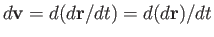. Thus,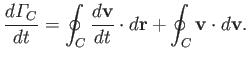(4.79)

By definition,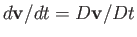for a co-moving loop. (See Section 1.10.) Moreover, the equation of motion of an incompressible inviscid fluid can be written [see Equation (1.79)]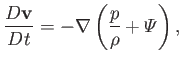(4.80)

because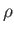is a constant. Hence,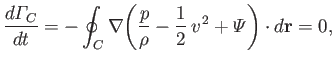(4.81)

because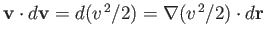(see Section A.18), and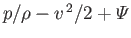is obviously a single-valued function.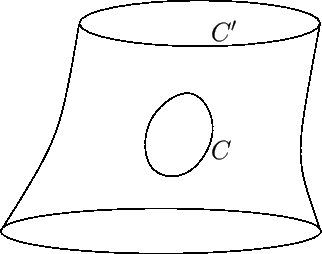One corollary of the Kelvin circulation theorem is that the fluid particles that form the walls of a vortex tube at a given instance in time continue to form the walls of a vortex tube at all subsequent times. To prove this, imagine a closed loopthat is embedded in the wall of a vortex tube but does not circulate around the interior of the tube. (See Figure 4.10.) The normal component of the vorticity over the surface enclosed byis zero, because all vorticity vectors are tangential to this surface. Thus, from Equation (4.77), the circulation around the loop is zero. By Kelvin's circulation theorem, the circulation around the loop remains zero as the tube is convected by the fluid. In other words, although the surface enclosed bydeforms, as it is convected by the fluid, it always remains on the tube wall, because no vortex filaments can pass through it.

Another corollary of the circulation theorem is that the intensity of a vortex tube remains constant as it is convected by the fluid. This can be proved by considering the circulation around the loop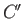pictured in Figure 4.10.Next: Irrotational Flow Up: Incompressible Inviscid Flow Previous: Circulation and Vorticity
Richard Fitzpatrick 2016-03-31